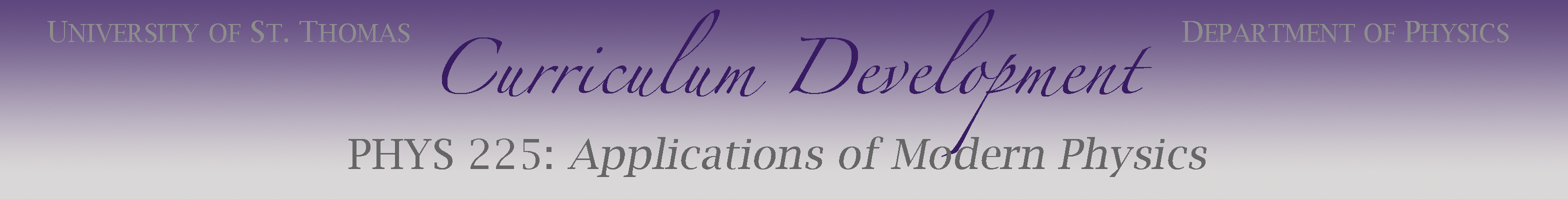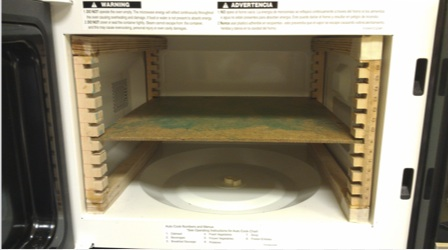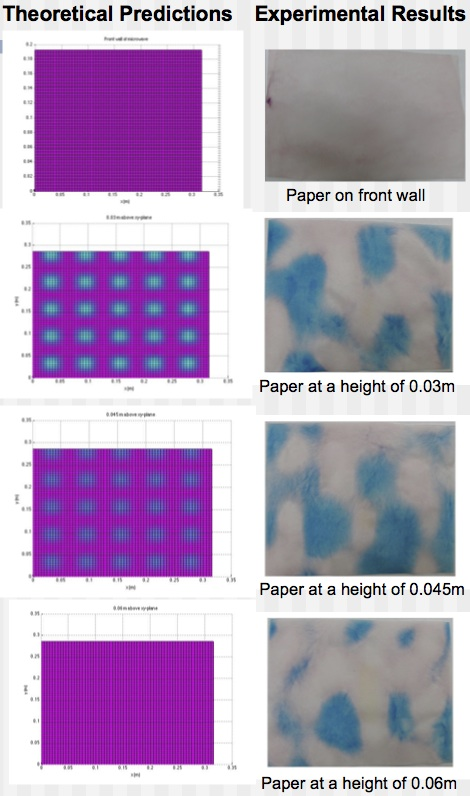## Lab #5 The Wave Equation

Conceptual goal: Become comfortable with the mathematical formalism of differential equations with boundary conditions. Understand how applying boundary conditions leads to quantization.
Experimental goal: Use the pattern of standing waves in a microwave oven to estimate the speed of light.

We have adapted the Wave Equations tutorial from the materials developed at the University of Colorado-Boulder . In the wave equation tutorial, students solve the wave equation for electromagnetic radiation inside a microwave oven, and find that the boundary conditions lead to only certain wavelengths being allowed (a first glimpse of quantization). The tutorial developed at UC-Boulder will be extended to include visualization of the stationary waves in two dimensions by plotting in Matlab. Students will use paper moistened with a CoCl2 solution to reveal the pattern of microwaves in an oven, following reference . They will then estimate the speed of light from the distance between nodes on the paper and the known operating frequency of the microwave.

Sample Results:### References:

1. S. B. McKagan, K. K. Perkins and C. E. Wieman, “Reforming a large lecture modern physics course for engineering majors using a PER-based design,” PERC Proceedings 2006, AIP Press. Melville, NY (2006). Website

2. A. Steyn-Ross and A. Riddell, “Standing waves in a microwave oven,” The Physics Teacher 28, 474 (1990).

This material is based upon work supported by the National Science Foundation under Grant Number DUE-1140034.
Any opinions, findings, and conclusions or recommendations expressed in this material are those of the author(s) and do not necessarily reflect the views of the National Science Foundation.